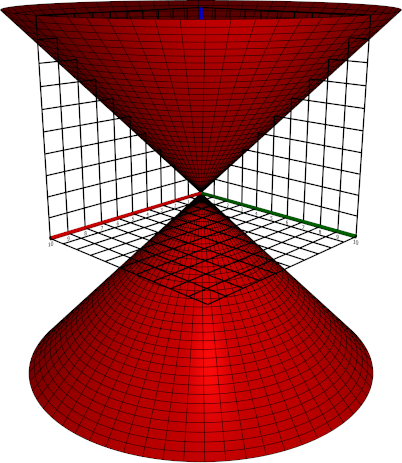$\newcommand{\sech}{\mathop{\rm sech}\nolimits} \newcommand{\csch}{\mathop{\rm csch}\nolimits} \newcommand{\R}{\mathbb{R}} \newcommand{\basis}{\underline{\mathbf{e}}}$
Current Page
Cone

# Cone

Object type: Surface

### Definition

In $\mathbb{R}^3$, a cone is a set of points $(x,y,z)$ satisfying the equation $$z^2 = \left(\frac{x}{a}\right)^2 + \left(\frac{y}{b}\right)^2$$ for some constants $a, b > 0$. Below the 'standard cone' $z^2 = x^2 + y^2$ is shown.Each intersection with a plane $z = c_0 \ne 0$ yields an ellipse, and therefore the surface is also called an elliptic cone. If $a = b$, each such intersection is a circle, and the surface is called a circular cone. Intersection with planes containing the $z$ axis yields a pair of lines through the origin; in particular, intersection with $y = 0$ yields the lines $z = \pm x/a, ~ y = 0$. In the case of a circular cone, the surface is the surface of revolution obtained as such a line is rotated about the $z$ axis.

### Parameterisation

The cone is the image $\mathbf{r}(\mathbb{R}\times\left[0,2\pi\right[)$ where $$\mathbf{r} (u,v) = \underline{\mathbf{e}}\begin{pmatrix}au\cos v\\bu\sin v\\u\end{pmatrix}.$$

### Properties

The following properties are with respect to the parameterisation given above.

#### Parameter-curve tangent vectors

The parameter-curve tangent vectors are $$\mathbf{r}_u(u,v) = \underline{\mathbf{e}}\begin {pmatrix}a\cos v\\b\sin v\\1\end{pmatrix}, \quad\quad \mathbf{r}_v(u,v) = \underline{\mathbf {e}}\begin{pmatrix}-au\sin v\\bu\cos v\\0\end{pmatrix}.$$

#### Standard unit normal

The standard unit normal vector field is $$\mathbf{\hat{N}}(u,v) = \frac{1}{\sqrt{b^2 u^2\cos^2 v + a^2 u^2 \sin^2 v + a^2 b^2 u^2 }}\underline{\mathbf{e}}\begin{pmatrix}-bu\cos v\\-au\sin v\\abu\end{pmatrix}$$ which, in the case of a circular cone, reduces to $$\mathbf{\hat{N}}(u,v) = \frac{\text{sgn}u}{\sqrt{1+a^2}} \underline{\mathbf{e}}\begin{pmatrix}-\cos v\\-\sin v\\a \end{pmatrix}.$$

#### Area element

The area element is $$dA = \sqrt{b^2 u^2\cos^2 v+ a^2 u^2 \sin^2 v + a^2 b^2 u^2}~dudv$$ which, in the case of a circular cone, reduces to $$dA = a|u|\sqrt{1+a^2}~dudv.$$

#### First fundamental form

The first fundamental form of the elliptic cone is $$\mathcal{F}(u,v) = \begin{pmatrix}a^2\cos^2 v +b^2\sin^2 v + 1&&(b^2-a^2)u\sin v\cos v\\(b^2-a^2)u\sin v\cos v&&a^2 u^2 \sin^2 v+b^2 u^2 \cos^2 v \end{pmatrix}$$ which, in the case of a circular cone, reduces to $$\mathcal{F}(u,v) = \begin{pmatrix} a^2+1&&0\\0&&a^2 u^2\end{pmatrix}.$$

#### Second fundamental form

The second fundamental form is $$\mathcal{M}(u,v) = \frac{abu^2}{\sqrt{b^2 u^2\cos^2 v + a^2 u^2 \sin^2 v + a^2 b^2 u^2 }}\begin{pmatrix}0&&0\\0&&1\end{pmatrix},$$ which, in the case of a circular cone, reduces to $$\mathcal{M}(u,v) = \frac{a|u|}{\sqrt{1+a^2}}\begin{pmatrix}0&&0\\0&&1 \end{pmatrix}.$$

#### Christoffel symbols

In the case of a circular cone ($a=b$), the Christoffel symbols are $$\Gamma^1_{\alpha\beta} = \frac{-a^2u}{a^2+1}\begin{pmatrix}0&&0\\0&&1\end{pmatrix}, \quad\quad \Gamma^2_{\alpha\beta} = \frac {1}{u}\begin{pmatrix}0&&1\\1&&0\end{pmatrix}.$$

#### Curvatures

In the case of a circular cone ($a=b$), the principal curvatures are $$\kappa_1 = 0, \quad\quad \kappa_2 = \frac{1}{a|u|\sqrt{1+a^2}}$$ with corresponding principal directions $(1,0)$ and $(0,1)$, respectively. Thus, the Gaussian and mean curvatures are $$K = 0, \quad\quad H = \frac{1}{a|u|\sqrt {1+a^2}}.$$